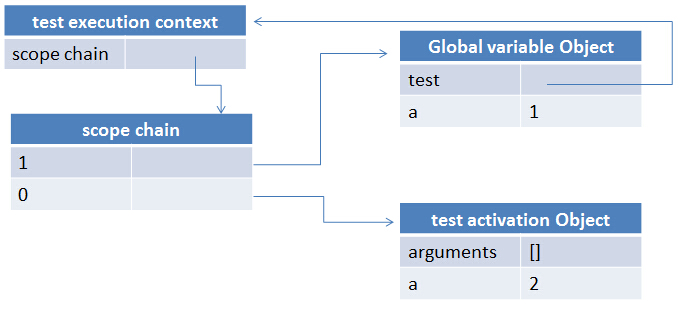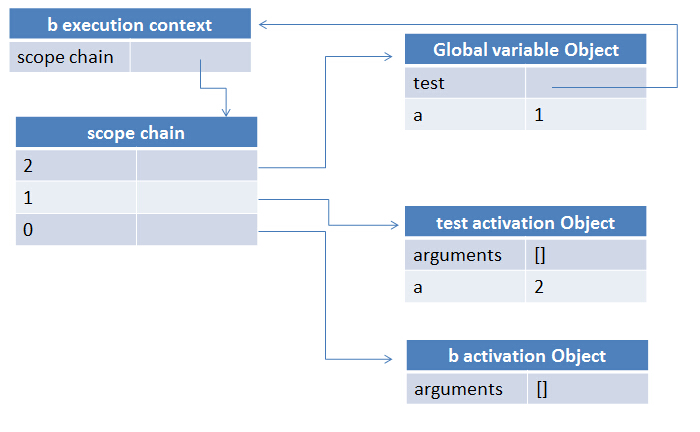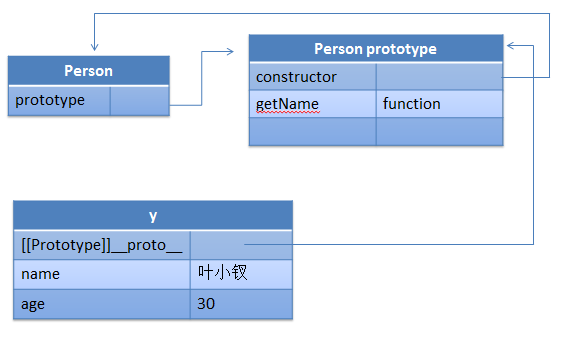# javascript中的一些核心知识点以及需要注意的地方

2400℃

javascript中，作用域链的作用是控制变量的访问顺序，仅此而已

 ```function test() { var a = 2; console.log(a); } var a = 1; test();```① 控制变量访问顺序

② 执行上下文包含一个作用域链的指针

③ 该层函数外部有几个函数，便会有几个活动对象待处理，作用域链指针会指向其外部活动对象

④ 作用域链为执行上下文时函数内部属性，不要妄想去操作

 ```function test() { var a = 2; return function () { console.log(a); }; } var b = test(); b();```

 `var b = test();````function createFn() { var ret = [], i; for (i = 0; i < 10; i++) { ret[i] = function () { return i; }; } return ret; } var fns = createFn();```

 ```function createFn() { var ret = [], i; for (i = 0; i < 10; i++) { ret[i] = (function (i) { return function () { return i; }; })(i); } return ret; } var fns = createFn();```

requireJS中的闭包

 ```define(function () { var add = function (x, y) { return x + y; }; return { add: add }; });```

webapp中的闭包

webapp一般会使用requireJS管理模块，而内部又会形成许多view的实例，这个实例并且会保存下来，这样也会导致很多函数的活动对象得不到释放```(function () { var Person = function (name) { this.name = name; }; //Person.prototype = {};//这句将影响十分具有constructor属性 Person.prototype.getName = function () { return this.name; }; var Student = function (name, sex, id) { this.name = name || '无名氏'; this.sex = sex || '不明'; this.id = id || '未填'; //学号 }; //相当于将其prototype复制了一次，若是包含constructor的话将指向Person Student.prototype = new Person(); Student.prototype.getId = function () { return this.id; } var y = new Person(); var s = new Student; var s1 = y instanceof Person; var s2 = s instanceof Student; var s3 = s instanceof Person; var s4 = Student.prototype.constructor === Person; var s5 = Student.constructor === Person; var s6 = Student.constructor === Function; var s = ''; })();```

 `Student.prototype = {}`

 ```var arr = []; var slice = arr.slice; function create() { if (arguments.length == 0 || arguments.length > 2) throw '参数错误'; var parent = null; //将参数转换为数组 var properties = slice.call(arguments); //如果第一个参数为类（function），那么就将之取出 if (typeof properties === 'function') parent = properties.shift(); properties = properties; function klass() { this.initialize.apply(this, arguments); } klass.superclass = parent; klass.subclasses = []; if (parent) { var subclass = function () { }; subclass.prototype = parent.prototype; klass.prototype = new subclass; parent.subclasses.push(klass); } var ancestor = klass.superclass && klass.superclass.prototype; for (var k in properties) { var value = properties[k]; //满足条件就重写 if (ancestor && typeof value == 'function') { var argslist = /^\s*function\s*\(([^\(\)]*?)\)\s*?\{/i.exec(value.toString()).replace(/\s/i, '').split(','); //只有在第一个参数为\$super情况下才需要处理（是否具有重复方法需要用户自己决定） if (argslist === '\$super' && ancestor[k]) { value = (function (methodName, fn) { return function () { var scope = this; var args = [function () { return ancestor[methodName].apply(scope, arguments); } ]; return fn.apply(this, args.concat(slice.call(arguments))); }; })(k, value); } } klass.prototype[k] = value; } if (!klass.prototype.initialize) klass.prototype.initialize = function () { }; klass.prototype.constructor = klass; return klass; }```

javascript中的DOM事件

PS：javascript的事件一块我说的够多了，这里再说一次吧……

javascript注册dom事件的手段很多：

① 直接写在dom标签上，onclick的做法

② 在js中这样写：el.onclick = function

① 我们事件是绑定到document上面，那么我怎么知道我现在是点击的什么元素呢

② 就算我能根据e.target获取当前点击元素，但是我怎么知道是哪个元素具有事件呢

③ 就算我能根据selector确定当前点击的哪个元素需要执行事件，但是我怎么找得到是哪个事件呢

 ```var arr = []; var slice = arr.slice; var extend = function (src, obj) { var o = {}; for (var k in src) { o[k] = src[k]; } for (var k in obj) { o[k] = obj[k]; } return o; }; function delegate(selector, type, fn) { var callback = fn; var handler = function (e) { //选择器找到的元素 var selectorEl = document.querySelector(selector); //当前点击元素 var el = e.target; //确定选择器找到的元素是否包含当前点击元素，如果包含就应该触发事件 /************* 注意，此处只是简单实现，实际应用会有许多判断 *************/ if (selectorEl.contains(el)) { var evt = extend(e, { currentTarget: selectorEl }); evt = [evt].concat(slice.call(arguments, 1)); callback.apply(selectorEl, evt); var s = ''; } var s = ''; }; document.addEventListener(type, handler, false); }```

 ```
parent
child

 ```var a = 0, b = 1; setInterval(function () { a = 1; }, 0) while (1) { //... b++; if(a == 1) break; }```

settimeout真正的的用法是：

① 延时请求，减少不必要的请求

② 需要过多的操作dom结构时，为了闭包浏览器假死，可以使用settimeout

 ```.on('touchend MSPointerUp pointerup', function(e){ if((_isPointerType = isPointerEventType(e, 'up')) && !isPrimaryTouch(e)) return cancelLongTap() // swipe if ((touch.x2 && Math.abs(touch.x1 - touch.x2) > 30) || (touch.y2 && Math.abs(touch.y1 - touch.y2) > 30)) swipeTimeout = setTimeout(function() { touch.el.trigger('swipe') touch.el.trigger('swipe' + (swipeDirection(touch.x1, touch.x2, touch.y1, touch.y2))) touch = {} }, 0) // normal tap else if ('last' in touch) if (deltaX < 30 && deltaY < 30) { tapTimeout = setTimeout(function() { var event = \$.Event('tap') event.cancelTouch = cancelAll touch.el.trigger(event) if (touch.isDoubleTap) { if (touch.el) touch.el.trigger('doubleTap') touch = {} } else { touchTimeout = setTimeout(function(){ touchTimeout = null if (touch.el) touch.el.trigger('singleTap') touch = {} }, 250) } }, 0) } else { touch = {} } deltaX = deltaY = 0 })```

localstorage

localstorage的使用在我厂webapp的应用中，达到了一个前所未有的高度，我们惊奇的发现，其真实容量是：

localstorage 的最大限制按字符数来算，中英文都是最多500多万个字符，webkit为5242880个

……